## Chapter 2. Creating Geometries

csGeometry is an abstract class. All derivations of the class represent one or more geometric objects, either concrete (such as a sphere or cube) or abstract (such as geoSet). The appearance of a shape—whether a sphere is dotted or striped— is characterized by a csAppearance object, csContext object, or both. Combining a geometry with an appearance completely describes the graphic content of a rendered object.

A csGeoSet is a collection of primitives, such as points, lines, triangles, and triangle strips, that, when arranged, create a geometry. For example, a collection of points can represent a star field and a collection of triangles can be arranged to form a sphere or a landscape.

After a brief terminology overview, the first part of this chapter discusses the ready-made geometries available in Cosmo 3D, such as csSphere and csCube. The remainder of the chapter discusses how to create your own csGeoSet-derived classes and how to use the csGeoSet-derived classes provided by Cosmo 3D.

These are the sections in this chapter:

## Geometry Terminology

Table 2-1 briefly summarizes the geometry terminology used in this manual. Understanding the key terms will help you understand the discussions of the different elements.

Table 2-1. Geometry Terminology

Term

Description

Encapsulated in

Geometry

An object of any form; the surface of which is uniform and non-descript.

csGeometry objects or objects derived from this class.

Appearance

Contains all the parameters that specify the look of a geometry.

csAppearance object.

Shape

Combination of a geometry and an appearance.

csShape object.

Context

Maintains and manages the graphics state.

csContext object.

## Using Large Geometries

• csSphere

• csCube

• csBox

• csCone

• csCylinder

Each class has methods that allow you to set and retrieve the values necessary to define the geometry, including (where appropriate)

• coordinates of the center

• height

• width

The names of the methods that set and retrieve these values are intuitively obvious, for example, to set and retrieve the coordinates of the center of a geometry, you use methods similar to the following:

 ```void setCenter(const csVec3f& center); void getCenter(csVec3f& center); ```

## Creating csGeoSet Objects

csGeoSet is a virtual class from which all geometric primitives are derived. Each csGeoSet-derived class contains a collection of primitives, such as points, quads, or triangle strips. All of the primitives in a collection are of the same type. You can construct a geometric object by specifying the coordinates of several of these primitives and combine them in a collection. For example, you can arrange triangles to form a sphere or a landscape. The vertices, normals, colors, and texture coordinates of each primitive are captured as attributes of each primitive.

Figure 2-1 illustrates how:

• Each csGeoSet-derived object contains an array of primitive shapes.

• Each primitive is made of an array of four attributes.

• Each of the four attributes refers to an array of attribute values, as shown in Figure 2-1.Note: The order of the attributes can be changed depending on the needs of the application.

Figure 2-1. Primitives in a csGeoSetThese attributes are captured in csGeoSet fields.

### csGeoSet Fields

The fields in a csGeoSet object can be grouped in the following manner:

Table 2-2. Fields in a csGeoSet

Field

Default

General settings

short cullFace
int primCount

BACK
0

Attribute specifications

Color colors
Normal normals
TexCoord texCoords
Coord coords

NULL
NULL
NULL
NULL

Attribute index specifications

Index colorIndices
Index normalIndices
Index texCoordIndices
Index coordIndices

NULL
NULL
NULL
NULL

Attribute binding specifications

char colorBind
char normalBind
char texCoordBind

OFF
OFF
OFF

The remainder of this section describes csGeoSet general settings. The other parts of this chapter describe the attribute fields.

### Setting the Number of Primitives

The following csGeoSet methods affect all of the primitives in a csGeoSet object:

 ```void setPrimCount(csInt primCount); csInt getPrimCount(); ```

To specify or retrieve the number of primitives in a csGeoSet, use the setPrimCount() and getPrimCount() methods. Appendix B, “Cosmo 3D Sample Application” shows how to retrieve the number of primitives in a csGeoSet.

## csGeoSet Attributes

csGeoSet is a virtual class from which all geometric primitives are derived. Cosmo 3D-supplied csGeoSet-derived classes include, for example:

• csPointSet—A collection of equally-sized points.

• csLineStripSet—A collection of linestrips, also known as polylines, of equal width.

• csTriStripSet—A collection of triangle strips.

• csPolySet—A collection of convex, coplanar polygons.

All of the primitives within a given set are equal in size. These primitives are defined by an array of four attributes:

• color—(red, green, blue, alpha)

• normal—(Nx, Ny, Nz)

• texture coordinates—(S, T)

• coordinates—(X, Y, Z)

Each attribute consists of an array of two to four values; a primitive is defined by these twelve values.Note: Although texture coordinates can be specified using four values (S, T, R, Q), the R value has no current meaning in Cosmo 3D because it does not support textures greater than two dimensions, and the Q value is always one.

### Attribute Bindings

Not all attributes can be applied with the same level of specificity. The levels of specificity include

• The entire collection of primitives in a csGeoSet object.

• Individual primitives in a csGeoSet object.

• Individual vertices of individual primitives in a csGeoSet object.

For example, a single color can be specified for the entire collection of primitives, for individual primitives, or per vertex. One set of coordinates, on the other hand, cannot be specified for the entire collection of primitives, cannot be specified for individual primitives, but must be specified per vertex. It does not make sense for all of the primitives in a collection to have the same coordinates, nor does it make sense for all vertices in each primitive to have the same coordinates. Each vertex must have its own coordinates.

Each level of specificity is called a different binding, for example, an attribute that is specified for an entire collection of primitives is said to have an OVERALL binding. A binding tells you how many primitives in a csGeoSet object an attribute applies to. Table 2-3 shows the different possible bindings.

Table 2-3. Attribute Bindings

OFF

OVERALL

PER_PRIMITIVE

PER_VERTEX

colors

yes

yes

yes

yes

normals

yes

yes

yes

yes

texture coordinates

yes

no

no

yes

coordinates

no

no

no

yes

All attributes in a csGeoSet collection must share the same set of attribute bindings, for example, you cannot specify colors-per-vertex for some primitives and colors-per-primitive for others in the same csGeoSet object, the color binding must be the same. You can, however, have, for example, color-per-vertex and overall normal bindings in the same csGeoSet.

#### Setting Attribute Bindings

Three set...() methods in csGeoSet specify the attribute bindings for a csGeoSet object:

 ```void setNormalBind(NormalBindEnum normalBind); void setColorBind(ColorBindEnum colorBind); void setTexCoordBind(TexCoordBindEnum texCoordBind); ```

There is a corresponding set of get...() methods that retrieve the attribute bindings for the normals, colors, and texture coordinates, respectively.

The enumerated binding values that are valid for each of the attributes coincide with the entries in Table 2-3.

 ```enum NormalBindEnum { NO_NORMS, OVERALL_NORMS, PER_PRIM_NORMS, PER_VERTEX_NORMS, }; enum ColorBindEnum { NO_COLORS, OVERALL_COLORS, PER_PRIM_COLORS, PER_VERTEX_COLORS, }; enum TexCoordBindEnum { NO_TEX_COORDS, PER_VERTEX_TEX_COORDS } ```

To set the color of all the primitives in a csGeoSet object to the same value, for example, use the OVERALL_COLORS binding in code similar to the following:

 ```csTriangleStripSet* myTriangleStrip = new csTriangleStripSet(); myTriangleStrip->setColorBind(csGeoSet::OVERALL_COLORS); ```

## Setting Attributes

Now that you know how to set attribute bindings, you need to know how to set the attributes themselves.

As shown in Figure 2-1, csGeoSet objects store their primitives in an array. The array contains:

• Three attribute values in the Normal array.

• Three (or four) attribute values in the Color array.

• Two attribute values in the Texture Coordinate array.

• Three attribute values in the Coordinate array.

This pattern continues, as shown in Figure 2-2.

Figure 2-2. Sequential Specification of Attributes Per Primitive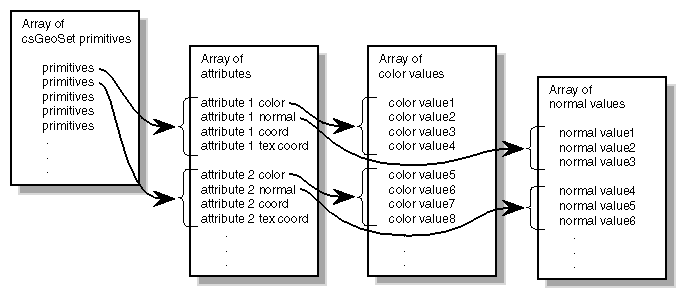### Indexing Attributes

Another option is to index the attribute values so that primitives can access any attribute value and more than one primitive can use the same attribute value, as shown in Figure 2-3.

Figure 2-3. Indexed Attributes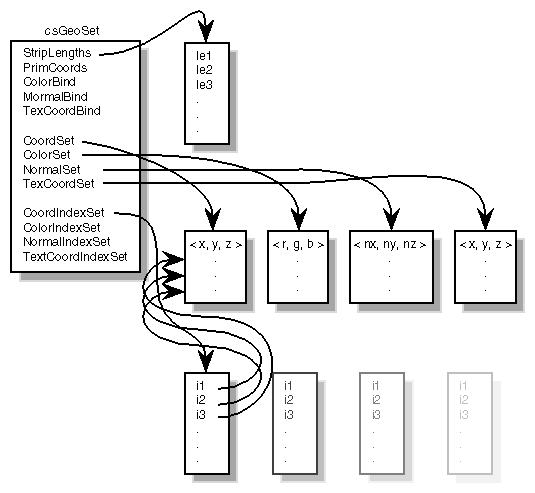#### When to Index Attributes

For all primitives in a csGeoSet, you have to decide whether to use indexed or sequential attributes; that is, all of the primitives within one csGeoSet must be referenced either sequentially or by index. You cannot mix the two reference methods.

The governing principle for indexing attributes or not is how many vertices in a geometry are shared. Consider the following two examples in Figure 2-4, where each dot marks a vertex.

Figure 2-4. Deciding Whether to Index Attributes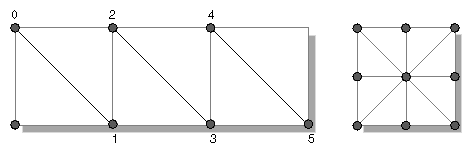In the triangle strip, each vertex is shared by two adjoining triangles. In the square, the same vertex is shared by eight triangles. Consider the task that is required to move these vertices when, for example, morphing the object. If the vertices were not indexed, in the square, the application would have to look up and alter eight triangles to change one vertex.

In the case of the square, it is much more efficient to index the attributes. On the other hand, if the attributes in the triangle strip were indexed, since each vertex is shared by only two triangles, the index look-up time would exceed the time it would take to simply update the vertices sequentially. In the case of the triangle strip, rendering is improved by handling the attributes sequentially.

The deciding factor governing whether or not to index attributes relates to the number of primitives that share the same attribute: if attributes are shared by many primitives, the attributes should be indexed; if attributes are not shared by many primitives, the attributes should be handled sequentially.

“Indexing Attributes” describes the methods you use to index attributes.

### Specifying Attributes

Whether you index your attributes or not, you must use the following set...() methods in csGeoSet to specify the attributes in a specific csGeoSet object:

 ```void setCoordsSet(csCoordSet* coords); void setNormalsSet(csNormalSet* normals); void setColorsSet(csColorSet* colors); void setTexCoordsSet(csTexCoordSet* texCoords); ```

There is a corresponding set of get...() methods that retrieve the index settings for the coordinates, normals, colors, and texture coordinates, respectively.

The set...() methods have the following arguments:

• coords is a three-dimensional array of coordinates representing the coordinates of every vertex in every primitive in a csGeoSet object.

• normals is a three-dimensional array of normals for potentially every vertex in every primitive in a csGeoSet object, depending on the binding.

• colors is a four-dimensional array of colors for potentially every vertex in every primitive in a csGeoSet object, depending on the binding.

• texCoords is a two-dimensional array of coordinates representing the texture coordinates of every vertex in every primitive in a csGeoSet object.

#### Using More Specific Attribute Arrays

Each of the four attributes has its own array. You must use one of the more specifically-defined virtual array classes, as follows:

 ```csCoordSet3f(); csNormalSet3f(); csColorSet3f(); csColorSet4f(); csTexCoordSet2f(); ```

Each of these null constructors is overridden by a set of two constructors that are similar in form to the following:

 ```csCoordSet3f(int n); csCoordSet3f(csData *array, short offset, short stride); ```

The first constructor allows you to specify the number of array primitives, n.

The second constructor allows you to reference an array, *array, of attribute values, specify the offset, offset, if any, and the stride, stride.

The stride mechanism that lets an application choose to keep all data staggered in a single array (or use two arrays). For example, you could combine color, vertex, and coordinate data and access each type as needed using the stride number. Stride specifies the byte offset between pointers to consecutive vertexes, in effect, stride is a relative offset, as shown in Figure 2-5.

Figure 2-5. Stride and Offset Values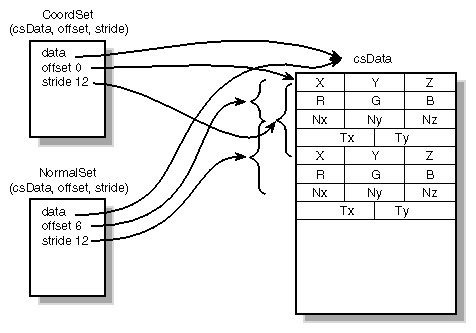Set and Get Methods

Each of the virtual attribute-array classes, both the general and specific, have set and get methods to set and return the values of the array. All set and get methods use the following form:

 ```void setCoordsSet(csCoordSet* coords); csCoordSet* getCoordsSet(); ```

#### Indexing Attributes

An indexed csGeoSet object uses a list of unsigned short integers to index an attribute array. Four set...() methods in csGeoSet specify these indices:

 ```void setCoordIndices(csIndexSet* coordIndices); void setNormalIndices(csIndexSet* normalIndices); void setColorIndices(csIndexSet* colorIndices); void setTexCoordIndices(csIndexSet* texCoordIndices); ```

There is a corresponding set of get...() methods that retrieve the index settings for the coordinates, normals, colors, and texture coordinates, respectively.

coordIndices is an array of coordinate indices. Each index points to a member in the coordinate attribute array, as shown in Figure 2-3.

normalIndices is an array of normal indices. colorIndices is an array of color indices. texCoordIndices is an array of texture coordinate indices.

### Setting Attributes Example

Example 2-1 shows how to set attributes and their bindings.

Example 2-1. Setting Attributes

 ```// Create a csGeoSet object csTriStripSet *gset = new csTriStripSet; // Allocate the attribute arrays csCoordSet3f *vset = new csCoordSet3f(NumRings*RingVerts); csNormalSet3f *nset = new csNormalSet3f(NumRings*RingVerts); csIndexSet *iset = new csIndexSet((NumRings-1) * 2 * (RingVerts + 1)); csColorSet4f *cset = new csColorSet4f(NumRings-1); csIndexSet *lengths = new csIndexSet(NumRings-1); // Set the attributes gset->setCoords(vset); gset->setNormals(nset); gset->setColors(cset); // Set the attribute indices gset->setCoordIndices(iset); gset->setNormalIndices(iset); gset->setPrimCount(NumRings-1); // Set the attribute bindings gset->setNormalBind(csGeoSet::PER_VERTEX_NORMS); gset->setColorBind(csGeoSet::PER_PRIM_COLORS); // Prepare to fill the Attribute and Indices arrays csVec3f *coords = vset->coords()->edit(); csVec3f *norms = nset->normals()->edit(); int *indices = iset->indices()->edit(); ```

### Editing Attribute Arrays

Cosmo 3D allows you to modify the values in arrays using the csNormalSet3f::edit() and csNormalSet3f::editDone() methods. Although you can modify the values, you cannot change the number of values in the array.

edit() returns a pointer to the attribute array. editDone() notifies any engines or sensors connected to this field that the array has changed.

It is illegal to call any other editing methods between edit() and editDone().

Example 2-2 shows an example of editing attribute arrays.

Example 2-2. Editing Attribute Arrays

 ```// cube normals csNormalSet3f *nset = new csNormalSet3f(numCubeNorms); nset->vector()->edit(); #if 0 for (i=0; ivector()->set(i, csVec3f(cubeNorms[i], cubeNorms[i], cubeNorms[i])); #else nset->vector()->setRange(0, numCubeNorms, (csVec3f *)cubeNorms); #endif nset->vector()->editDone(); gset->setNormalSet(nset); ```

## Cosmo 3D-Derived csGeoSet Objects

Cosmo 3D provides the following csGeoSet collections. Each is a derivative of csGeoSet.

• csPointSet—A collection of equally-sized points.

• csLineSet—A collection of lines of equal length.

• csIndexedLineSet—A set of indexed line strips.

• csLineStripSet—A collection of linestrips, also known as polylines.

• csTriSet—A collection of triangles.

• csTriFanStrip—A collection of triangles that share a common vertex.

• csTriStripSet—A collection of triangle strips.

• csPolySet—A collection of convex, coplanar polygons.

• csIndexedFaceSet—A polygon with faces that are indexed.

The following sections describe each of these primitive collections.

All of the classes contain virtual draw() and calcBound() methods. The draw() method specifies how a csGeoSet object is drawn. The calcBound() method specifies how the bounding box is computed. Other fields are specific to their geometries.

### Using csPointSet

A csPointSet object contains a collection of equally-sized points. Point size is the diameter of each point in pixels.

csPointSet contains the following fields:

 ```void  setSize(csFloat size); csFloat getSize(); ```

The setSize() and getSize() methods allow you to specify and find out, respectively, the diameter, in pixels, of all the points in a csPointSet object.

### Using csLineSet

A csLineSet object contains a collection of lines of equal length. The fields allow you to set and return the width of the lines used for drawing.

 ```void setWidth(csFloat width); csFloat getWidth(); ```

### Using csIndexedLineSet

A csIndexedLineSet object contains an indexed collection of lines of equal length. The fields allow you to set and return the colors of the lines in the collection.

 ```csMFInt* coordIndex() const; csMFInt* colorIndex() const; void setColorPerVertex(csBool colorPerVertex); csBool getColorPerVertex(); ```

### Using csLineStripSet

A csLineStripSet object contains a collection of linestrips, otherwise known as polylines, of equal width. Line width is specified in pixels.

csLineStripSet contains the following fields:

 ```csMFInt* stripLength () const void setWidth(csFloat width); csFloat getWidth(); ```

TheStripLength() method allows you to specify and find out, respectively, how many line segments are in a csLineStripSet object.

The setWidth() and getWidth() methods allow you to specify and find out, respectively, the width, in pixels, of each linestrip in a csLineStripSet object.

### Using csTriSet

A csTriSet object contains a collection of triangles. This class serves as a class from which csTriFanSet and csTriStripSet are derived.

### Using csTriFanSet

A csTriFanSet is a set of triangles all of which share one common vertex, as shown in Figure 2-6.

Figure 2-6. TriFanSet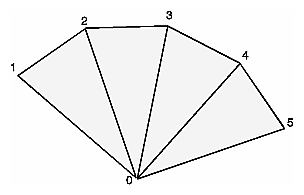You use the following method to retrieve or set the number of triangles in the csTriFanSet.

 ```csMFInt* fanLength() const; ```

### Using csTriStripSet

A csTriStripSet object contains a collection of triangle strips. A triangle strip is a series of adjacent triangles that form a strip, as shown in Figure 2-7.

Figure 2-7. Triangle Strip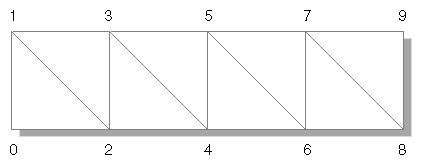csTriStripSet contains the following field:

 ```csMFInt* stripLength() const; ```

This field allows you to specify and find out how long each triangle strip is in a csTriStripSet object. The length is expressed in the number of vertices per strip, for example, three tristrips with individual lengths of 4, 6, and 8, would be represented by an array of three integers:

 ```csMFInt* length = [4, 6, 8]; ```

### Using csPolySet

A csPolySet object contains a collection of polygons. Polygons may have different numbers of sides but must be convex and coplanar.

csPolySet contains the following methods:

 ```csMFInt* polyLength () const; ```

This field allows you to specify and find out how many sides there are per polygon in a csPolySet object.

### Using csIndexedFaceSet

A csIndexedFaceSet object contains a collection of polyhedrons of equal size. The member functions allow you to set and return the size of the polyhedrons in the collection.

 ```csMFInt* coordIndex() const; csMFInt* colorIndex() const; csMFInt* normalIndex() const; csMFInt* texCoordIndex() const; void setCCW(csBool ccw); void setSolid(csBool solid); void setConvex(csBool convex); void setCreaseAngle(csFloat creaseAngle); void setColorPerVertex(csBool colorPerVertex); void setNormalPerVertex(csBool normalPerVertex); ```

There is a corresponding get...() method for every set...() statement.

The first four fields contain arrays for storing the color.

setCCW() is true if the vertices of these faces wind counter-clockwise when viewed from the front.

setSolid() is true if this set of faces forms a closed volume (“solid”); in that case, faces on the side of the solid facing away from the viewpoint don't need to be drawn.

setConvex() is true if the faces in this set are convex. (Currently ignored.)

setCreaseAngle() sets the crease angle. If the angle between two faces is more than the crease angle, the faces are assumed to be part of a single surface and are smooth shaded. (Currently ignored.)

coordIndex() sets a VRML 2.0-style vertex coordinate index set.

colorIndex() sets a VRML 2.0-style color index set.

texCoordIndex() sets a VRML 2.0-style texture coordinate index set.

normalIndex() sets a VRML 2.0-style normal index set.

setColorPerVertex() is true if colors are assigned per vertex, otherwise per face.

setNormalPerVertex() is true if normals are assigned per vertex, otherwise per face.